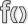# Plot.GetArea (Civ5 API)

Jump to: navigation, search

This page is a part of the Lua and UI Reference (Civ5).This function is a member of Plot. This is an instance method, invoke it with a colon.

# Usage

`AreaID Plot:GetArea()`

Returned Value

No description available.

# Source code samples

Redundant occurences have been removed.

Amazon_XP.lua (G&K)

`DLC/Expansion/Maps/Amazon_XP.lua`
 `0987` `local area = plot:GetArea()` `0989` `-- Adding this check for Great Plains` `0990` `if x < 1 or x >= iW - 1 or y < 1 or y >= iH - 1 then`

AssignStartingPlots.lua (G&K)

`DLC/Expansion/Gameplay/Lua/AssignStartingPlots.lua`
 `0888` `local thisPlotsArea = plot:GetArea()` `0889` `if thisPlotsArea ~= iAreaID then -- This plot is not a member of the landmass, set value to 0` `1554` `local iArea = plot:GetArea();` `1791` `local area_of_plot = plot:GetArea();` `5225` `local iAreaID = plot:GetArea();` `6328` `local area = plot:GetArea()` `6329` `if area ~= area_ID and area_ID ~= -1 then`

Boreal.lua (G&K)

`DLC/Expansion/Maps/Boreal.lua`
 `0718` `local area = plot:GetArea()` `0720` `-- Adding this check for Highlands` `0721` `if x < 1 or x >= iW - 1 or y < 1 or y >= iH - 1 then`

Europe.lua (G&K)

`DLC/Expansion/Maps/Europe.lua`
 `0492` `local testAreaID = testPlot:GetArea()` `0493` `local testArea = Map.GetArea(testAreaID)`

FeatureGenerator.lua (G&K)

`DLC/Expansion/Gameplay/Lua/FeatureGenerator.lua`
 `0382` `local iArea = adjPlot:GetArea()` `0383` `local adjArea = Map.GetArea(iArea)`

Four_Corners.lua

`Maps/Four_Corners.lua`
 `0498` `local area = plot:GetArea()` `0500` `-- Adding this check for Four Corners` `0501` `if x < 1 or x >= iW - 1 or y < 1 or y >= iH - 1 then`

InlandSea.lua

`Maps/InlandSea.lua`
 `0302` `local area = plot:GetArea()` `0304` `-- Adding this check for Inland Sea` `0305` `if x < 1 or x >= iW - 1 or y < 1 or y >= iH - 1 then`

Lakes.lua

`Maps/Lakes.lua`
 `0194` `local area = plot:GetArea()` `0196` `-- Adding this check for Lakes` `0197` `if y < 4 or y >= iH - 4 then`

MapGenerator.lua (G&K)

`DLC/Expansion/Gameplay/Lua/MapGenerator.lua`
 `0626` `local areaID = plot:GetArea();`

MapmakerUtilities.lua

`Gameplay/Lua/MapmakerUtilities.lua`
 `0098` `local area = plotFirst:GetArea();` `0102` `area = plotLast:GetArea();` `0138` `local area = plot:GetArea();`

North_vs_South.lua

`Maps/North_vs_South.lua`
 `0669` `local area = plot:GetArea()` `0671` `-- Adding this check for North vs South` `0672` `if x < 1 or x >= iW - 1 or y < 1 or y >= iH - 1 then`

Ring.lua

`Maps/Ring.lua`
 `0642` `local area = plot:GetArea()` `0644` `-- Adding this check for Ring, to force all City States to the polar region in the center.` `0645` `if (x > centWestX and x < centEastX and y > centSouthY and y < centNorthY) == false then`

Skirmish.lua

`Maps/Skirmish.lua`
 `1067` `local area = plot:GetArea()` `1069` `-- Adding this check for Skirmish.` `1070` `if x < 1 or x >= iW - 1 or y < 1 or y >= iH - 1 then`

Terra.lua

`Maps/Terra.lua`
 `0650` `local adjAreaID = adjPlot:GetArea();` `0749` `local iAreaID = adjPlot:GetArea();`

West_vs_East.lua

`Maps/West_vs_East.lua`
 `0569` `local area = plot:GetArea()` `0571` `-- Adding this check for West vs East.` `0572` `if x < 1 or x >= iW - 1 or y < 1 or y >= iH - 1 then`

The initial version of this page was created by the Civ5 API Bot, see the Civ5 API Reference FAQ. Some of the texts come from the 2kgames' wiki and most of code samples are copyrighted to Firaxis.
Functions' signatures were either copied from the 2kgames' wiki, or infered from the Lua source files and the binaries. Errors are possible.
Contributors may find help in the Contributors guide to the Civ5 API.